Courses

# Mixtures and Allegations - Examples (with Solutions), Arithmetic, Quantitative Reasoning Quant Notes | EduRev

## Banking Exams : Mixtures and Allegations - Examples (with Solutions), Arithmetic, Quantitative Reasoning Quant Notes | EduRev

The document Mixtures and Allegations - Examples (with Solutions), Arithmetic, Quantitative Reasoning Quant Notes | EduRev is a part of the Banking Exams Course Quantitative Aptitude (Quant).
All you need of Banking Exams at this link: Banking ExamsBasics of Fractions OR Chain Rule

• When a fraction has its numerator greater than the denominator, its value is greater than one. Let us call it greater fraction. Whenever a number (say x) is multiplied by a greater fraction, it gives a value greater than itself.
• When a fraction has its numerator less than the denominator, its value is less than one. Let us call it less fraction. Whenever a number (say x) is multiplied by a less fraction, it gives a value less than itself.

➢ Rule of Fractions OR Chain Rule

Let us try to understand the concept with the help of an example.

Example.1 If 6 men can do a piece of work in 30 days of 9 hours each, how many men will it take to do 10 times the amount of work if they work for 25 days of 8 hours?

Three points arise:

• Less days, so more men required.
• Less working hours, so more men required.
• More work, more men.

By rule of fraction or chain rule:

Step I: We look for our required unit. It is the number of men. So, we write down the number of men given in the question. It is 6.
Step II: The number of days gets reduced from 30 to 25, so it will need more men (Reasoning: Less days, more men). It simply means that 6 should be multiplied by a greater fraction because we need a value greater than 6. So, we have: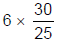Step III: Following in the same way, we see that the above figure should be multiplied by a ‘greater fraction’, i.e., by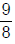. So, we have: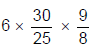Step IV: Following in the same way, we see that the above figure should be multiplied by a ‘greater fraction’ i.e. by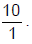So, we have: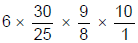= 81 men.

Mixtures & Alligation

• As the dictionary meaning of Alligation (mixing), we will deal with problems related to mixing of different compounds or quantities. The concept of alligation and weighted average are the same.
• When two or more quantities are mixed together in different ratios to form a mixture, then ratio of the quantities of the two constituents is given by the following formulae: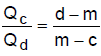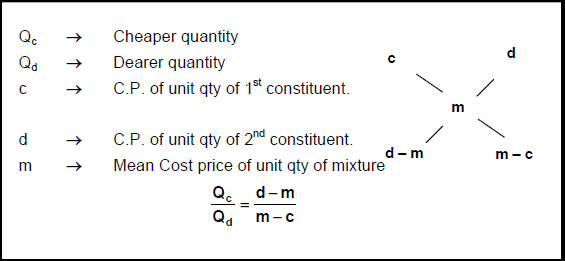• Gives us the ratio of quantities in which the two ingredients should be mixed to get the mixture.

Example.2 A sum of Rs 39 was divided among 45 boys and girls. Each girl gets 50 paise, whereas a boy gets one rupee. Find the number of boys and girls.

• Average amount of money received by each =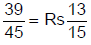• Amount received by each girl = 50 paise = Rs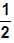• Amount received by each boy = Re. 1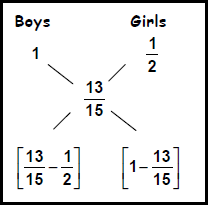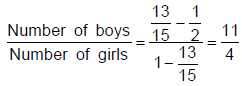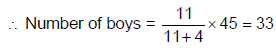Number of girls = 45 – 33 = 12.

Important Funda

• Always identify the ingredients as cheaper & dearer to apply the alligation rule.
In the alligation rule, the variables c, d & m may be expressed in terms of percentages (e.g. A 20% mixture of salt in water), fractions (e.g. two-fifth of the solution contains salt) or proportions (e.g. A solution of milk and water is such that milk : Water = 2 : 3).
• The important point is to remember is that c & d may represent pure ingredients or mixtures.

(Mixing a pure component into a solution)
Example.3 A jar contains a mixture of two liquids A and B in the ratio 4 : 1. When 10 litres of the liquid B is poured into the jar, the ratio becomes 2: 3. How many litres of liquid A were contained in the jar?

Method 1: (weighted average or equation method)

• Let the quantities of A & B in the original mixture be 4x and x litres.
• According to the question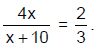12x = 2x + 20
⇒ 10x = 20
⇒ x = 2
• The quantity of A in the original mixture = 4x = 4 × 2 = 8 litres.

Method 2: (Alligation with composition of B)

• The average composition of B in the first mixture is 1/5.
• The average composition of B in the second mixture = 1
• The average composition of B in the resultant mixture = 3/5
• Hence applying the rule of Alligation we have
[1 – (3/5)]/[(3/5) – (1/5)] = (2/5)/(2/5) = 1
So, initial quantity of mixture in the jar = 10 litres.
And, quantity of A in the jar = (10 × 4)/5 = 8 litres.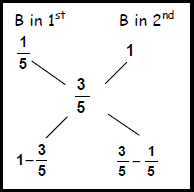Method 3: (Alligation with percentage of B)

• The percentage of B in 1st mixture = 20%
• The percentage of B in 2nd mixture = 100%
• The percentage of B in Final Mixture = 60%
• By rule of allegation we have
Volume 1st : Volume 2nd = (100% - 60%) : (60% - 20%)
V1 : V2 = 1 : 1
Volume of mixture 1st = 10 litres
Volume of A in mixture 1st = 80% of 10 litres = 8 litres. Answer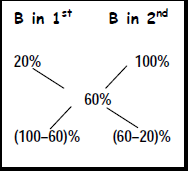Try yourself:A vessel is filled with liquid, 3 parts of which are water and 5 parts syrup. How much of the mixture must be drawn off and replaced with water so that the mixture may be half water and half syrup?

Try yourself:Tea worth Rs. 126 per kg and Rs. 135 per kg are mixed with a third variety in the ratio 1 : 1 : 2. If the mixture is worth Rs. 153 per kg, the price of the third variety per kg will be:

➢ Removal and Replacement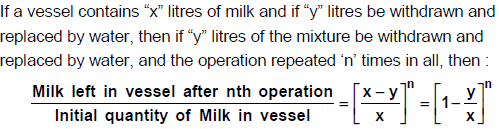Example.4 Nine litres of solution are drawn from a cask containing water. It is replaced with a similar quantity of pure milk. This operation is done twice. The ratio of water to milk in the cask now is 16 : 9. How much does the cask hold?

• Let there be x litres in the cask
• After n operations:(1 – 9/x)2 = 16/25
∴ x = 45 litres.

Example.5 There are two containers A and B of milk solution. The ratio of milk and water in container A is 3 : 1 and in container B, it is 4 : 1. How many liters of container B solution has to be added to 20. lts of container ‘A’ solution such that in the resulting solution; the ratio of milk to water should be 19 : 6?

• In container A, the part of milk =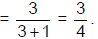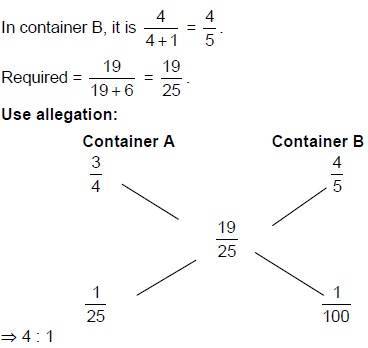• It is given 20 lts of container A is added. So, the quantity of container B should be 5 lts.

Example.6 There are two alloys A and B. Alloy A contains zinc, copper and silver, as 80% 15% and 5% respectively, whereas alloy B also contains the same metals with percentages 70%, 20%, 10% respectively. If these two alloys are mixed such that the resultant will contain 8% silver, what is the ratio of these three metals in the resultant alloy?

• Since the resultant alloy contains 8% silver, first we will find, in what ratio these two alloys A and B were mixed to form the resultant.
• Then the resultant zinc percentage is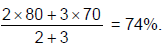• So, copper percentage = 100 – (74 + 8) = 18
∴ The ratio of these metals = 74 : 18 : 8 = 37 : 9 : 4.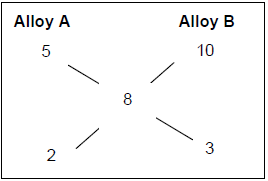Example.7 The cost of an apple is directly proportional to square of its weight in a fruit bazaar. Two friends A and B went there to purchase apples. A got exactly 5 apples per kg and each apple is of same weight. Where as B got exactly 4 apples per kg each weight is exactly same. If B paid Rs. 10 more than A per kg apples, what is the cost of an apple which weighs 1 kg?

• It is given cost ∝ (weight)2
⇒ c = k w2.
• A got 5 apples per kg and each apple is of same weight. ⇒ Each apple is 200 gm. = 1/5 kg.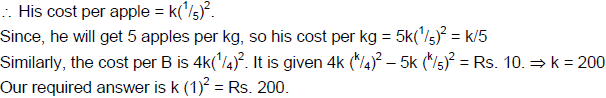Offer running on EduRev: Apply code STAYHOME200 to get INR 200 off on our premium plan EduRev Infinity!

## Quantitative Aptitude (Quant)

134 videos|131 docs|131 tests

,

,

,

,

,

,

,

,

,

,

,

,

,

,

,

,

,

,

,

,

,

,

,

,

,

,

,

;